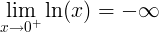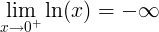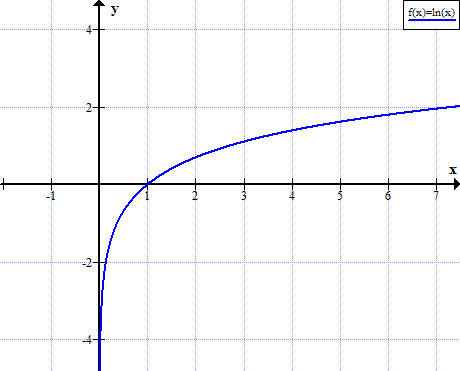# Natural logarithm

ln(x) = loge(x) = y

The e constant or Euler's number is:

e ≈ 2.71828183

## Ln as inverse function of exponential function

The natural logarithm function ln(x) is the inverse function of the exponential function ex.

For x>0,

f (f -1(x)) = eln(x) = x

Or

f -1(f (x)) = ln(ex) = x

## Natural logarithm rules and properties

Rule name Rule Example

#### Product rule

ln(x ∙ y) = ln(x) + ln(y)

ln(37) = ln(3) + ln(7)

#### Quotient rule

ln(x / y) = ln(x) - ln(y)

ln(3 / 7) = ln(3) - ln(7)

#### Power rule

ln(x y) = y ∙ ln(x)

ln(28) = 8ln(2)

#### ln derivative

f (x) = ln(x) f ' (x) = 1 / x

#### ln integral

ln(x)dx = x ∙ (ln(x) - 1) + C

#### ln of negative number

ln(x) is undefined when x ≤ 0

#### ln of zero

ln(0) is undefinedln(1) = 0

#### ln of infinity

lim ln(x) = ∞ ,when x→∞
Euler's identity ln(-1) = iπ

#### Logarithm product rule

The logarithm of the multiplication of x and y is the sum of logarithm of x and logarithm of y.

logb(x ∙ y) = logb(x) + logb(y)

For example:

log10(37) = log10(3) + log10(7)

#### Logarithm quotient rule

The logarithm of the division of x and y is the difference of logarithm of x and logarithm of y.

logb(x / y) = logb(x) - logb(y)

For example:

log10(3 / 7) = log10(3) - log10(7)

#### Logarithm power rule

The logarithm of x raised to the power of y is y times the logarithm of x.

logb(x y) = y ∙ logb(x)

For example:

log10(28) = 8log10(2)

#### Derivative of natural logarithm

The derivative of the natural logarithm function is the reciprocal function.

When

f (x) = ln(x)

The derivative of f(x) is:

f ' (x) = 1 / x

#### Integral of natural logarithm

The integral of the natural logarithm function is given by:

When

f (x) = ln(x)

The integral of f(x) is:

f (x)dx = ∫ ln(x)dx = x ∙ (ln(x) - 1) + C

#### Ln of 0

The natural logarithm of zero is undefined:

ln(0) is undefined

The limit near 0 of the natural logarithm of x, when x approaches zero, is minus infinity:#### Ln of 1

The natural logarithm of one is zero:

ln(1) = 0

#### Ln of infinity

The limit of natural logarithm of infinity, when x approaches infinity is equal to infinity:

lim ln(x) = ∞, when x→∞

## Complex logarithm

For complex number z:

z = re = x + iy

The complex logarithm will be (n = ...-2,-1,0,1,2,...):

Log z = ln(r) + i(θ+2nπ) = ln(√(x2+y2)) + i·arctan(y/x))

## Graph of ln(x)

ln(x) is not defined for real non positive values of x:## Natural logarithms table

x ln x
0 undefined
0+ - ∞
0.0001 -9.210340
0.001 -6.907755
0.01 -4.605170
0.1 -2.302585
1 0
2 0.693147
e ≈ 2.7183 1
3 1.098612
4 1.386294
5 1.609438
6 1.791759
7 1.945910
8 2.079442
9 2.197225
10 2.302585
20 2.995732
30 3.401197
40 3.688879
50 3.912023
60 4.094345
70 4.248495
80 4.382027
90 4.499810
100 4.605170
200 5.298317
300 5.703782
400 5.991465
500 6.214608
600 6.396930
700 6.551080
800 6.684612
900 6.802395
1000 6.907755
10000 9.210340

Rules of logarithm »

Currently, we have around 1976 calculators, conversion tables and usefull online tools and software features for students, teaching and teachers, designers and simply for everyone.